# Using Kotlin in Android Studio 3.0 (Part 4)

### In Part 4, we'll continue learning about object-oriented programming for Kotlin, looking at interface and data classes.

· Mobile Zone · Tutorial
Save
4.28K Views

In Part 3, I introduced some aspects of object-oriented programming (OOP) for Kotlin. In this article, I will continue to introduce other aspects of OOP.

## Interface

We can use interfaces the way we are used in Java. A Kotlin interface method can have a default implementation. Unlike Java 8, which requires the  `default ` keyword, Kotlin has no special annotation for such methods: you just provide a method body. Example:

``````interface Person {
fun canWork():String = "I can unknown!"
}``````

Kotlin interfaces can have properties but these need to be abstract or to provide accessor implementations. Example:

``````interface Person {
var Name:String //abstract
var Gender:String // abstract
fun canWork():String = "I can unknown!"
}``````

We can implement interfaces in a class look like this:

``````class Student:Person
{
override var Name:String=""
get() = field.toUpperCase()
set(value){
field="I am \$value"
}
override var Gender:String =""
var StudentID:String = ""
var University:String=""
override fun canWork(): String {
return "\$Name .I am learning at \$University. My ID is \$StudentID and my gender is \$Gender \n"
}

}``````

Kotlin doesn’t support multiple inheritances, however, the same thing can be achieved by implementing more than two interfaces at a time.

## Data Classes

Like most other aspects of Kotlin, data classes aim to reduce the amount of boilerplate code you write in your project. Data classes provide compiler-generated  `equals()` , ` hashCode()` ,  `toString()` ,  `copy()` , and other methods. An example of a normal class:

``````class Person {
var Name: String = ""
}``````

We create an object from the  `Person ` class:

``````val p = Person()
println( p.toString())``````

The result can look like this:

`` …Person@5281e6c0 ``

Now, we change the  `Person ` class to become a data class by using  `data ` keyword as follows:

``data class Person(var Name:String = "")``

We create an object from the Person class again:

``````val p = Person(Name="Minh")
println( p.toString())``````

The result can look like this:

``Person(Name=Minh)``

If we declare some properties inside  `Person ` class body as follows:

``````data class Person1(var Name:String =""){
var Gender:String=""
}``````

Creating an object

``````val p = Person(Name="Minh")
p.Gender ="Male"
println( p.toString())``````

The result also looks like this:

``Person(Name=Minh)``

This is because the compiler only uses the properties defined inside the primary constructor for  `toString()` ,  `equals()` ,  `hashCode()` , and `copy()` implementations.

We also can use the `copy()` method copy an object altering some of its properties. An example:

``````val person = Person(Name="Minh", Gender="Male",Address = "NewYork")
val otherperson = person.copy(Address = "Chicago")
println( p.toString()) // The result: Person(Name=Minh,Gender=Male,Address=Chicago)``````

### Nested and Inner Classes

In Kotlin, classes can be nested in other classes:

``````class FlyAnimal {
//…..
class Bird {
//…
}
}``````

A class may be marked as  `inner ` to be able to access members of outer class. Inner classes carry a reference to an object of an outer class:

``````class FlyAnimal {
private val wings: Int = 2
inner class Bird {
var birdWings = wings
}
}
println (FlyAnimal().Bird().birdWings.toString()) // 2``````

### Sealed Classes

When you evaluate an expression using the  `when ` construct, the Kotlin compiler forces you to check for the default option. Example:

``````interface Expr
class Num(val value: Int) : Expr
class Sum(val left: Expr, val right: Expr) : Expr
fun eval(e: Expr): Int =
when (e) {
is Num -> e.value
is Sum -> eval(e.right) + eval(e.left)
else -> throw IllegalArgumentException("Unknown expression")
}``````

If you add a new subclass, the compiler won’t detect that something has changed. If you forget to add a new branch, the default one will be chosen, which can lead to subtle bugs. Kotlin provides a solution to this problem: : sealed classes. Let’s look at the following example:

``````sealed class Expr {
class Num(val value: Int) : Expr()
class Sum(val left: Expr, val right: Expr) : Expr()
}
fun eval(e: Expr): Int =
when (e) {
is Expr.Num -> e.value
is Expr.Sum -> eval(e.right) + eval(e.left)
}``````

If you handle all subclasses of a sealed class in a when statement, you don’t need to provide the default branch.

## An Android Application

In this application, I created an UI as follows: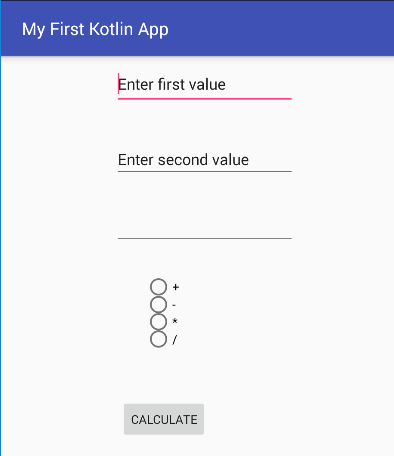We can input the first value, the second value, and choose an operator as follows: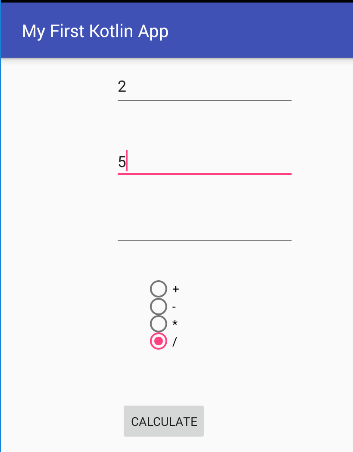Click the  `CALCULATE ` button, the result can look like this: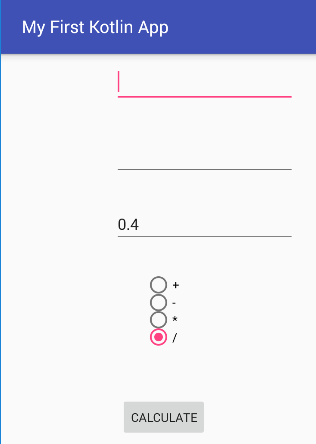Some controls are used in this application:

(You can see source code of activity_main.xml and strings.xml files here)

In the MainActivity.kt, the first, I created the sealed class named `Expr` and its subclasses:

``````sealed class Expr {
class Num(val value: Double) : Expr()
class Add(val left: Expr, val right: Expr) : Expr()
class Sub(val left: Expr, val right: Expr) : Expr()
class Mul(val left: Expr, val right: Expr) : Expr()
class Div(val left: Expr, val right: Expr) : Expr()
}``````

In MainActivity class, I created the  `eval ` function:

``````fun eval(e: Expr): Double =
when (e) {
is Expr.Num -> e.value
is Expr.Add -> eval(e.left) + eval(e.right)
is Expr.Sub -> eval(e.left) - eval(e.right)
is Expr.Mul -> eval(e.left) * eval(e.right)
is Expr.Div -> eval(e.left) / eval(e.right)
}``````

In OnClickListener, I got some inputs, assigned them to variables, and checked if a string is numeric or not using regular expressions (regex):

``````var numeric = true
str1 = value1.text.toString()
str2 = value2.text.toString()
//Check if a string is numeric or not using regular expressions (regex)
numeric = str1.matches("-?\\d+(\\.\\d+)?".toRegex()) && str2.matches("-?\\d+(\\.\\d+)?".toRegex())
if(numeric){
Val1 = value1.text.toString().toDouble()
Val2 = value2.text.toString().toDouble()
}
else{
Val1 = 0.0
Val2 = 0.0
}``````

The finally, I wrote some code:

``````// choose an operator
if(sub.isChecked)
Result = eval(Expr.Sub(Expr.Num(Val1), Expr.Num(Val2)))
if(mul.isChecked)
Result = eval(Expr.Mul(Expr.Num(Val1), Expr.Num(Val2)))
if(div.isChecked)
Result = eval(Expr.Div(Expr.Num(Val1), Expr.Num(Val2)))
// display result
ResultDisplay = String.format("%.1f", Result)
result.setText(ResultDisplay)
value1.text.clear()
value2.text.clear()``````

I also didn’t forget to set  `Click ` event for the  `CALCULATE ` button:

``````val buttonClickListener = View.OnClickListener { view ->
//…
}
calculate.setOnClickListener(buttonClickListener)``````

Run application again: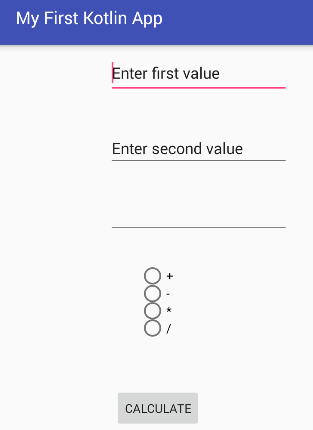You input texts for the first value and second value as follows: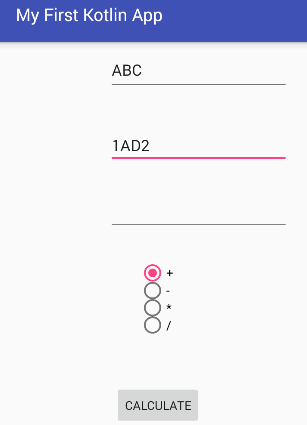Choose an operator and click the  `CALCULATE ` button, the result looks like this: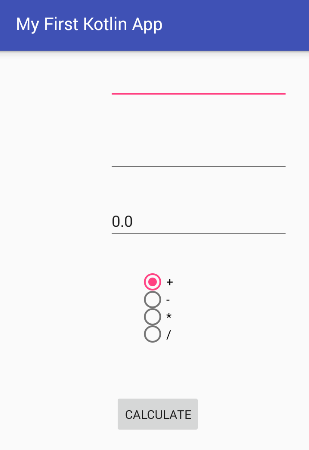## Conclusion

You can download my source here and I hope you have a great experience.

Topics:
android, reactive programming, mobile, mobile app development, tutorial

Opinions expressed by DZone contributors are their own.